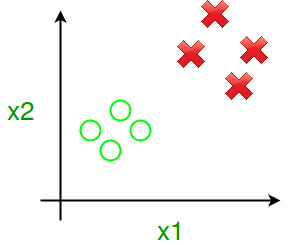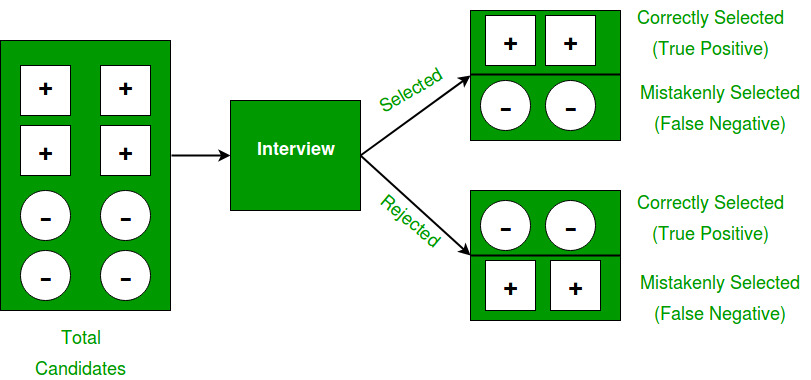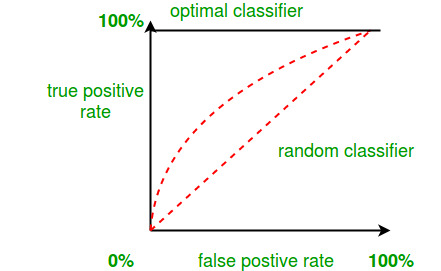Open In App

# Calculate Efficiency Of Binary Classifier

Prerequisite: Getting started with Classification

In this article, we will discuss a method to calculate the efficiency of a Binary classifier. Let’s assume there is a problem where we have to classify a product that belongs to either class A or class B.Let us define few statistical parameters :

TP (True Positive) = number of Class A products, which are classified as Class A products.
FN (False Negative) = number of Class A products, which are classified as Class B products.
TN (True Negative) = number of Class B products, which are classified as Class B products.
FP (False Positive) = number of Class B products, which are classified as Class A products.

```FP = N-TP;      // where number N is the number of class A type products
FN = M-TN;     // where number M is the number of class B type products```

We shall look at this example, to understand these parameters well.

If (+) denotes fit candidates for Job and (-) denotes unfit candidates for Job.To calculate the Efficiency of the classifier we need to compute values of Sensitivity, Specificity, and Accuracy.

Sensitivity measures the proportion of positives that are correctly identified as such.
Also known as True positive rate(TPR).

Specificity measures the proportion of negatives that are correctly identified as such.
Also known as True negative rate(TNR).

Accuracy measures how well the test predicts both TPR and TNR.

```Sensitivity = ( TP / (TP+FN) ) * 100;
Specificity = ( TN/(TN+FP) ) * 100;
Accuracy = ( (TP+TN) / (TP+TN+FP+FN) ) * 100;
Efficiency = ( Sensitivity + Specificity + Accuracy ) / 3; ```

Let’s take the above example and compute the efficiency of selection :

Say fit candidates belong to class A and unfit candidates belong to class B.

```Before Interview :  N = 4 and M = 4

After Interview :
TP = 2
TN = 2
FP = N - TP = 2
FN = M - TN = 2

Sensitivity = 2/(2+2)*100 = 50
Specificity = 2/(2+2)*100 = 50
Accuracy    = (2+2)/(2+2+2+2)*100 = 50
Efficiency  = (50+50+50)/3 = 50

So,Efficiency of selection of candidates is 50% accurate.```

Other performance measures:

• Error rate = (FP + FN) / (TP + TN + FP + FN)
• Precision = TP / (TP + FP)
• Recall = TP / (TP + FN)
• BCR (Balanced Classification Rate) = 1/2* (TP / (TP + FN) + TN / (TN + FP))
• AUC = Area under ROC curve